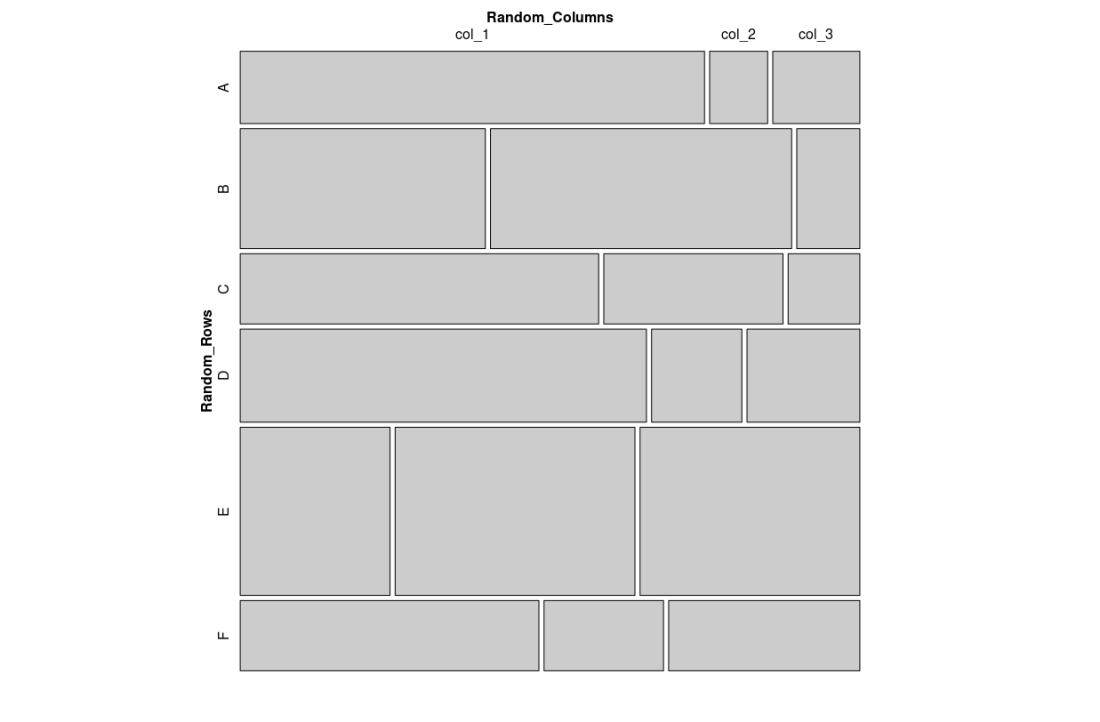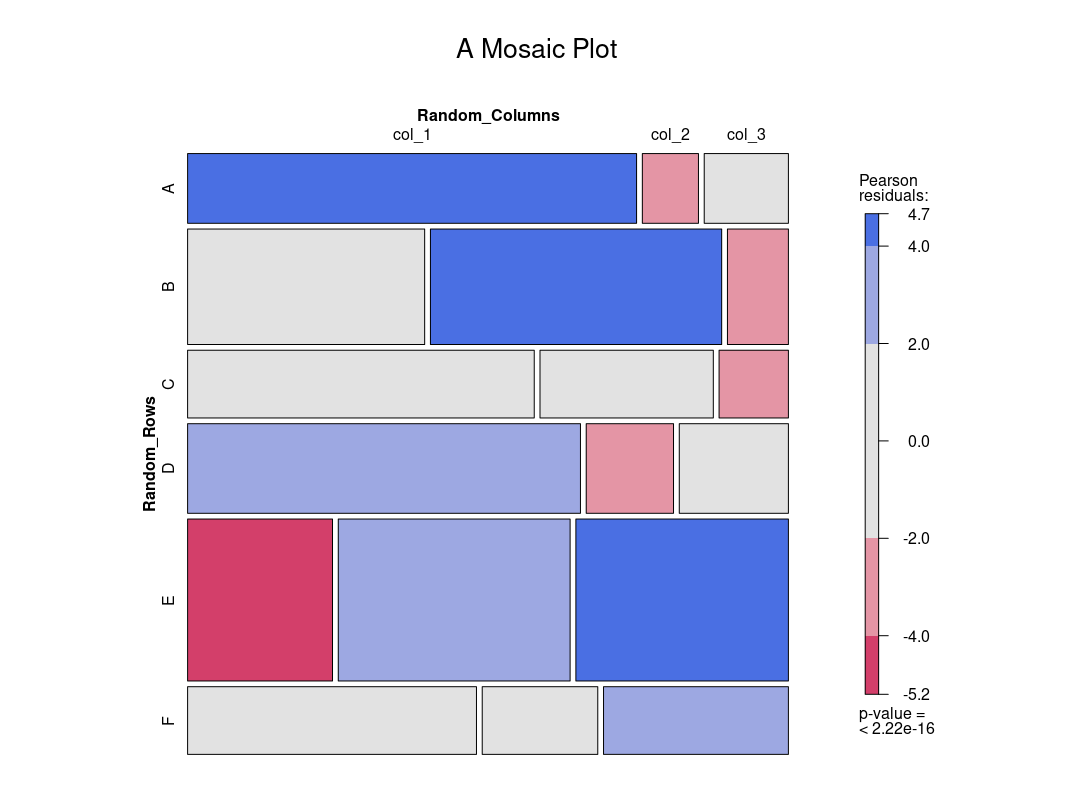# Mosaic Plot in R Programming

• Last Updated : 30 Jun, 2021

Mosaic Plots are used to show symmetries for tables that are divided into two or more conditional distributions. Mosaic plots are a great way to visualize hierarchical data. A collection of rectangles represents all the elements to be visualized with the rectangles of different sizes and colors makes a table, but what makes these mosaic charts unique is the arrangement of the elements where there is a hierarchy those elements are collected and labeled together, perhaps even with subcategories. So mosaic plots can be used for plotting categorical data very effectively, with the area of the data showing the relative proportions.

In this article, we will learn how to create a mosaic plot in R programming language. The package that is used for this is vcd.

### Method 1: Using mosaic() function

The function used for creating a mosaic plot in R programming language is mosaic().

Syntax:

Parameters:

• x: Here, x is pointing to the variable that holds the dataset/table. We passed our dataset name here.
• shade: shade is a boolean variable, if it is set to be true then we will get a colored plot. Its default value is NULL.
• legend: the legend is a boolean variable, if it is set to be true then we will be able to see legends alongside our mosaic plot. Its default value is NULL.
• main: main is a string variable, here we pass the title of our mosaic plot.

To create a plot, first the package is loaded into space, and the dataset is created. The created dataset is passed to the function.

Example 1:

## R

 `library``(``'vcd'``)`` ` `# creating a random dataset ``# creating 6 rows``data_values <- ``matrix``(``c``(80, 10, 15,``                        ``70, 86, 18, ``                        ``60, 30, 12,``                        ``90, 20, 25,``                        ``60, 96, 88, ``                        ``50, 20, 32))`` ` `# creating dataset with above values``data <- ``as.table``(``  ``matrix``(``    ``data_values,``     ` `    ``# specifying the number of rows``    ``nrow = 6,``    ``byrow = ``TRUE``,``     ` `    ``# creating two lists one for rows``    ``# and one for columns``    ``dimnames = ``list``(``      ``Random_Rows = ``c``(``'A'``,``'B'``,``'C'``, ``'D'``, ``'E'``, ``'F'``),``      ``Random_Columns = ``c``(``'col_1'``, ``'col_2'``, ``'col_3'``)``      ``)``    ``)``  ``)`` ` `# plotting the mosaic chart``mosaic``(data)`

Output:simple mosaic plot

A mosaic plot can also be drawn with custom features to make it more presentable.

Example 2:

## R

 `library``(``'vcd'``)`` ` `# creating a random dataset ``# creating 6 rows``data_values <- ``matrix``(``c``(80, 10, 15,``                        ``70, 86, 18, ``                        ``60, 30, 12,``                        ``90, 20, 25,``                        ``60, 96, 88, ``                        ``50, 20, 32))`` ` `# creating dataset with above values``data <- ``as.table``(``  ``matrix``(``    ``data_values,``     ` `    ``# specifying the number of rows``    ``nrow = 6,``    ``byrow = ``TRUE``,``     ` `    ``# creating two lists one for rows``    ``# and one for columns``    ``dimnames = ``list``(``      ``Random_Rows = ``c``(``'A'``,``'B'``,``'C'``, ``'D'``, ``'E'``, ``'F'``),``      ``Random_Columns = ``c``(``'col_1'``, ``'col_2'``, ``'col_3'``)``      ``)``    ``)``  ``)`` ` `# plotting the mosaic chart``mosaic``(data,``        ` `       ``# shade is used to plot colored chart``       ``shade=``TRUE``,``        ` `       ``# adding title to the chart``       ``main = ``"A Mosaic Plot"``)`

Output:A simple mosaic plot

My Personal Notes arrow_drop_up